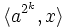# Elementary abelian-to-normal replacement fails for Klein four-group

(diff) ← Older revision | Latest revision (diff) | Newer revision → (diff)
This article discusses a failure of replacement, i.e., a situation where the analogue of a valid replacement theorem fails to hold under slightly modified conditions.
View other failures of replacement | View replacement theorems

## Statement

### Hands-on statement

We can find a finite group whose order is a power of$2$, that contains a Klein four-group (?) as a subgroup(i.e., it contains an elementary abelian subgroup of order four) but does not contain any normal subgroup that is a Klein four-group.

In fact, the following stronger statement is true: for any$k$, we can find a finite group whose order is a power of$2$, that contains a Klein four-subgroup but does not contain a$k$-subnormal Klein four-subgroup.

### Statement in terms of the weak normal replacement condition

The single-element collection$\mathcal{S}$ comprising the Klein four-group is not a Collection of groups satisfying a weak normal replacement condition (?) for the prime$2$.

## Proof

### Example of the dihedral group (for the weaker version)

Further information: dihedral group:D16, Subgroup structure of dihedral group:D16

Consider the dihedral group of order sixteen:$G := \langle a,x \mid a^8 = x^2 = 1, xax = a^{-1} \rangle$.

Then:

• The subgroup$\langle a^4, x \rangle$ is a Klein four-group.
• There is no normal Klein four-subgroup: Any subgroup contained in$\langle a \rangle$ is cyclic, so it cannot be a Klein four-group. Thus, a normal Klein four-subgroup, if it exists, must contain some element outside$\langle a \rangle$. However, the sizes of the conjugacy classes of elements outside$\langle a \rangle$ is$4$ each, so any normal subgroup containing an element outside$\langle a \rangle$ must have order strictly bigger than four.

### Example of the dihedral group (for the stronger version)

Further information: Dihedral group, Subgroup structure of dihedral groups

We need to take a dihedral group of order$2^{k+3}$, as follows:$G := \langle a,x \mid a^{2^{k+2}} = x^2 = 1, xax = a^{-1} \rangle$.

Then:

• The subgroup$\langle a^{2^{k+1}},x \rangle$ is a Klein four-group.
• There is no$k$-subnormal Klein four-subgroup: Any subgroup contained in$\langle a \rangle$ is cyclic, so it cannot be a Klein four-group. Thus, a$k$-subnormal Klein four-subgroup, if it exists, must contain some element outside$\langle a \rangle$. Since all elements outside$\langle a \rangle$ are in the same automorphism class, we can assume that it contains$x$. However, the smallest$k$-subnormal subgroup containing$x$, computed by taking the normal closure$k$ times, is a dihedral group of order eight given by$\langle a^{2^k},x \rangle$.